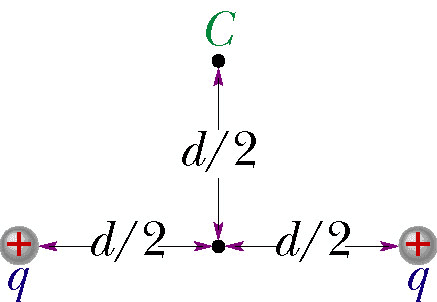# Two charges, Electrical Potential

## Homework StatementTwo charges q = 9.0μ C are fixed in space a distance d = 1.5 cm apart, as shown in the figure.

a) With V = 0 at infinity, what is the electric potential at point C?
b) You bring a third charge q = 9.0μC from infinity to C. How much work must you do?
c) What is the potential energy U of the three-charge configuration when the third charge is in place?

## Homework Equations

electrical potential=kq/r
μ=E-6
q=9E9

## The Attempt at a Solution

I thought that I should find the distance between q and C using the pythagorean theorem.
((9E9)(9.0E-6)/(2.1213 cm))+((9E9)(9.0E-6)/(2.1213 cm))=7.64E4 V
But this didn't work. The online homework said it was wrong.
Is that the right equation to use? How does the V=0 at infinity affect this problem?

TSny
Homework Helper
Gold Member
What's the SI unit for distance?

•rlc
meters
Was I right to calculate the distance from q to C? Is converting cm to m the only mistake?

TSny
Homework Helper
Gold Member
meters
Was I right to calculate the distance from q to C? Is converting cm to m the only mistake?
Yes. Yes No - See post below.

Last edited:
TSny
Homework Helper
Gold Member
Note that the legs of the triangle are d/2, not d.

•rlc
Wow, I really need to learn to read these diagrams!
That worked, thank you!

Part b asks: You bring a third charge q = 9.0μC from infinity to C. How much work must you do?

TSny
Homework Helper
Gold Member
Work is closely related to energy.

The work done by the electric field in moving an electric charge from infinity to point r is
given by:
W= - deltaU= - q(deltaV)= - q(Vr-Vinfinity)= - qVr

I found this equation online. Does it look like the right one to try?

TSny
Homework Helper
Gold Member
That's close. One of the annoying things about this topic is that when you calculate work, you have to be clear whether you're talking about the work done by the person moving the charge or the work done by the electric force. The force exerted by the person counteracts the electric force, so the work done by the person has opposite sign of the work done by the electric force. So, you need to consider whether the formula you are using is for the work done by the electric force or for the work done by the person.

I'm hoping its work done by electric force :)

TSny
Homework Helper
Gold Member
I'm hoping its work done by electric force :)
Yes, the formula you wrote is for the work done by the electric force. So, you have to modify it to get the work that you do in moving the charge to C.

Alright, so I figured it out!
Work=qV
where V is the number I just calculated for the first part of this question and q is the 3rd charge given
So, Work=(9.0E-6)(1.53E7V)=1.37E2 J

Then for part c, it's asking for the potential energy of the three charge configuration when the 3rd charge is in place, which the equation for is:
(work done)+(kq^2)/d
(1.37E2 J) + (9E9)(9.0E-6)^2/(0.015 m) = 1.86E2 J

Thank you for helping me thru this and finding my mistakes! I really appreciate it!